# Modelling Black Holes and Singularities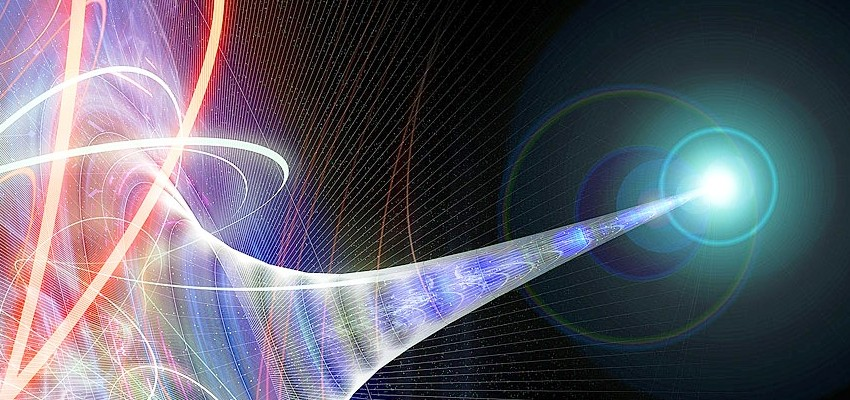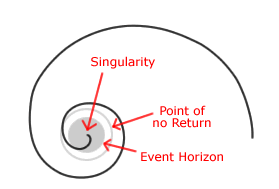Falling into a gravitational singularity presents a difficult challenge when animating the laws of physics. Although not discussing quite the same aspect of motion, Richard Feynman in The Character of Physical Law (1965) sums things up

It always bothers me that according to the laws as we understand them today, it takes a computing machine an infinite number of logical operations to figure out what goes on in no matter how tiny a region of space and no matter how tiny a region of time …

Modelling relativity with an extra inverse cubed factor on the distance (force on M₁ is G*M₂/D² + iRel*M₂/D³) rather then the normal Newtonian inverse square law (force on M₁ as G*M₂/D²) provided an easy way to introduce precession in the orbit of planets like Mercury. It also led to some complex orbits with fast precession, where objects got close to, but not inside, the “point of no return” of a black hole. Unfortunately, this style of animation does not properly track particles once you are inside the point of no return.

What calculations between frames should be used to model the path of an object falling into a black hole singularity?

For inspiration and a chance to make sense of what is going on, let’s take a look at the fundamental differences between Einstein’s gravity and Newton’s gravity.

#### Einstein’s Gravity and Newton’s Gravity

A note from Newton’s Principles definition #4 in 1687 gives Newton’s view of force

Impressed Force – This force conflicts in the action only; and remains no longer in the body when the action is over.

In Einstein’s second paper on relativity in 1905, he explicitly concludes

Radiation carries inertia between emitting and absorbing bodies.

It is important that not only does an object receive a “kick” from the momentum of the energy, but the internal inertia (i.e., the inertial mass) of the object is actually increased.

#### Elastic and Inelastic Collisions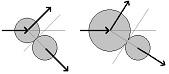An example of an elastic collision is where objects with given sizes collide and bounce off each other. They continue on as two independent objects. An inelastic collision means the objects are stuck together and continue on as if they were only one object.

Clearly an object falling into a black hole does not bounce off the black hole or fly out the other side. The particle somehow falls into the black hole and gets stuck inside. With Einstein’s gravity, we are modelling an inelastic collision

#### Modelling Attraction and Repulsion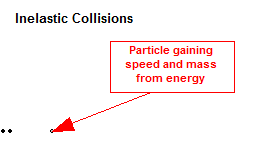Lets start with a model of repulsion and build on the idea that radiation carries inertia between emitting and absorbing bodies. Imagine shooting a continuous stream of bullets from a gun at an object floating in space. Not only are the bullets hitting the object and forcing it to go faster, but the bullets are getting stuck in the object, making it ever heavier and heavier. Clearly the object will never go faster then the bullets hitting it. Also note, the faster the object is going, the less effective hitting it with a bullet is, since the object is so much bigger and heavier.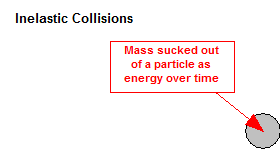To model attraction, we just reverse the time. Now imagine sucking bullets out of an object floating in space at the same speed you shot them in. The object would be attracted to you and over time, would be drained of its mass. Here again, the mass cannot quite get to the speed of the bullets, as all the mass will be pulled out of the object before it hits the speed of the bullets.

#### Modelling Einstein’s Gravity

It turns out that this model of attraction provides a very easy and straight forward way to build an animation of an object falling into a black hole. We simply subtract the amount of force that an object feels (expressed as mass) from the amount of mass an object already has. We add this same amount of mass to the other object.

In programming terms, between each frame, we calculate the force each object M1 feels from every other M2 object and update the masses

Force[M1] = Force[M1] + G*Mass[M2]/D² + iRel*Mass[M2]/D³
Mass[M1] = Mass[M1] – Force[M1]
Mass[M2] = Mass[M2] + Force[M1]

Followed by the standard frame update for each object M1

Momentum[M1] = Momentum[M1] + Force[M1]
Location[M1] = Location[M1] + Momentum[M1]

This now gives our object a very nice geodesic towards the singularity, together with the added benefit (if the calculation accuracy is high enough), that before the object hits the singularity, it will have the value of its mass go to zero.

As a bit of a sidebar here, this presents an opportunity to consider a slightly modified version of the usual visualization of the inside of a black hole. Instead of seeing the black hole as a big glob of nothingness, consider the black hole to be a huge mass of sloshing energy that cannot get out because of the gravitational pull.

Thanks for reading and considering these ideas. Coming soon: The quantum mechanical modelling of electrons and protons. What happens when an electron gets closer and closer to a proton?